# Beautiful boxplots and sassy small multiples¶

Author: Olga Botvinnik

## Beautiful boxplots¶

To depict a range of values for either categorical or sequential data, you've probably used boxplots, as below. We're going to improve this boxplot,

In :
import brewer2mpl
import random
import pandas as pd
import numpy as np

np.random.seed(10)

df = pd.DataFrame(np.random.randn(8, 4), columns=['A', 'B', 'C', 'D'])

set1 = brewer2mpl.get_map('Set1', 'qualitative', 7).mpl_colors

fig = plt.figure()

bp = ax.boxplot(df.values)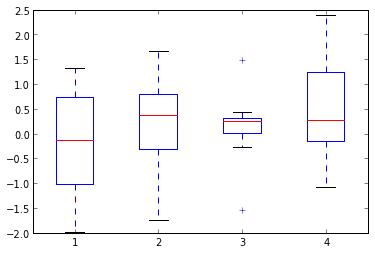According to this paper, boxplots are the most visually accurate way to depict this sort of data, compared to the "mid-gap" plots by Edward Tufte. The lack of boxes around the data obscures the quantiles and prevents comprehension.Image from http://matthiasklaus.girlshopes.com/chartjunkdesigninggoodgraphs/

While I do very much like Tufte's plots, I have to acknowledge that I want my plots to be easily comprehendable, so I will continue to use boxplots, but with a little tweaking.

So we'll just do some minor changes to clean up the graph, without removing the boxes. As from my previous tutorial, we removed the top, bottom and right axis lines of the plot via,

ax.spines['top'].set_visible(False)
ax.spines['right'].set_visible(False)
ax.spines['bottom'].set_visible(False)
In :
import random
import pandas as pd
import numpy as np

np.random.seed(10)

df = pd.DataFrame(np.random.randn(8, 4), columns=['A', 'B', 'C', 'D'])

fig = plt.figure()

bp = ax.boxplot(df.values)

ax.spines['top'].set_visible(False)
ax.spines['right'].set_visible(False)
ax.spines['bottom'].set_visible(False)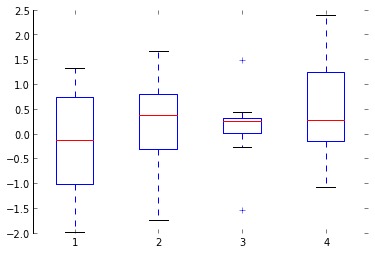And we also removed all the ticks via,

ax.xaxis.set_ticks_position('none')
ax.yaxis.set_ticks_position('none')
In :
import random
import pandas as pd
import numpy as np

np.random.seed(10)

df = pd.DataFrame(np.random.randn(8, 4), columns=['A', 'B', 'C', 'D'])

fig = plt.figure()

bp = ax.boxplot(df.values)

ax.spines['top'].set_visible(False)
ax.spines['right'].set_visible(False)
ax.spines['bottom'].set_visible(False)

ax.xaxis.set_ticks_position('none')
ax.yaxis.set_ticks_position('none')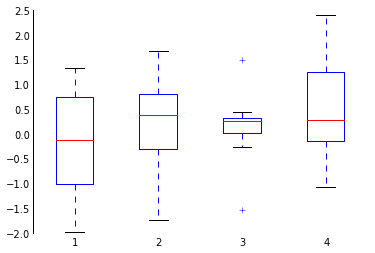Let's make sure we label the x-axis with,

ax.xaxis.set_ticklabels(df.columns)
In :
import random
import pandas as pd
import numpy as np

np.random.seed(10)

df = pd.DataFrame(np.random.randn(8, 4), columns=['A', 'B', 'C', 'D'])

fig = plt.figure()

bp = ax.boxplot(df.values)

ax.spines['top'].set_visible(False)
ax.spines['right'].set_visible(False)
ax.spines['bottom'].set_visible(False)

ax.xaxis.set_ticks_position('none')
ax.yaxis.set_ticks_position('none')

ax.xaxis.set_ticklabels(df.columns)

Out:
[<matplotlib.text.Text at 0x176ec6310>,
<matplotlib.text.Text at 0x11e14aa10>,
<matplotlib.text.Text at 0x115f050d0>,
<matplotlib.text.Text at 0x115f05450>]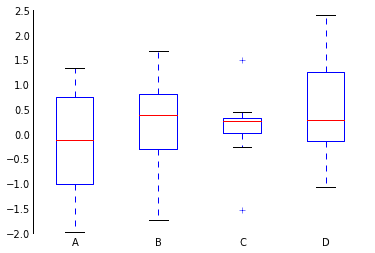This looks pretty nice, but let's change the blue and red to be the blue and red from 'Set1' instead of the default blue/red, via,

import brewer2mpl
set1 = brewer2mpl.get_map('Set1', 'qualitative', 7).mpl_colors

# Red
set1

# Blue
set1



Now what exactly do we want to change? From the matplotlib.pyplot.boxplot documentation, we can change these things,

• boxes: the main body of the boxplot showing the quartiles and the median’s confidence intervals if enabled.
• medians: horizonal lines at the median of each box.
• whiskers: the vertical lines extending to the most extreme, n-outlier data points.
• caps: the horizontal lines at the ends of the whiskers.
• fliers: points representing data that extend beyone the whiskers (outliers).

The syntax for changing these things is, plt.setp(bp['boxes'], **kwargs), and we're going to change the colors of each of these:

plt.setp(bp['boxes'], color=set1)
plt.setp(bp['medians'], color=set1)
plt.setp(bp['whiskers'], color=set1)
plt.setp(bp['fliers'], color=set1)
plt.setp(bp['caps'], color=set1)
In :
import brewer2mpl
import random
import pandas as pd
import numpy as np

np.random.seed(10)

df = pd.DataFrame(np.random.randn(8, 4), columns=['A', 'B', 'C', 'D'])

fig = plt.figure()

bp = ax.boxplot(df.values)

ax.spines['top'].set_visible(False)
ax.spines['right'].set_visible(False)
ax.spines['bottom'].set_visible(False)

ax.xaxis.set_ticks_position('none')
ax.yaxis.set_ticks_position('none')

ax.xaxis.set_ticklabels(df.columns)

set1 = brewer2mpl.get_map('Set1', 'qualitative', 7).mpl_colors
plt.setp(bp['boxes'], color=set1)
plt.setp(bp['medians'], color=set1)
plt.setp(bp['whiskers'], color=set1)
plt.setp(bp['fliers'], color=set1)
plt.setp(bp['caps'], color=set1)

Out:
[None, None, None, None, None, None, None, None]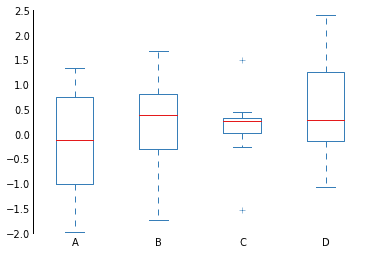This looks nice, but let's narrow the boxes via

bp = ax.boxplot(df.values, widths=0.15)
In :
import brewer2mpl
import random
import pandas as pd
import numpy as np

np.random.seed(10)

df = pd.DataFrame(np.random.randn(8, 4), columns=['A', 'B', 'C', 'D'])

set1 = brewer2mpl.get_map('Set1', 'qualitative', 7).mpl_colors

fig = plt.figure()

bp = ax.boxplot(df.values, widths=0.15)

ax.spines['top'].set_visible(False)
ax.spines['right'].set_visible(False)
ax.spines['bottom'].set_visible(False)

ax.xaxis.set_ticks_position('none')
ax.yaxis.set_ticks_position('none')

ax.xaxis.set_ticklabels(df.columns)

set1 = brewer2mpl.get_map('Set1', 'qualitative', 7).mpl_colors
plt.setp(bp['boxes'], color=set1)
plt.setp(bp['medians'], color=set1)
plt.setp(bp['whiskers'], color=set1)
plt.setp(bp['fliers'], color=set1)
plt.setp(bp['caps'], color=set1)

Out:
[None, None, None, None, None, None, None, None]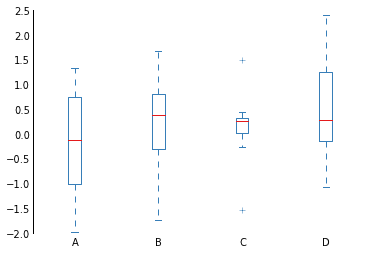I'm not a fan of the dashed lines here. Let's make the whiskers solid lines, but thin, with this command,

plt.setp(bp['whiskers'], color=set1, linestyle='solid', linewidth=0.5)
In :
import brewer2mpl
import random
import pandas as pd
import numpy as np

np.random.seed(10)

df = pd.DataFrame(np.random.randn(8, 4), columns=['A', 'B', 'C', 'D'])

set1 = brewer2mpl.get_map('Set1', 'qualitative', 7).mpl_colors

fig = plt.figure()

bp = ax.boxplot(df.values, widths=0.15)

ax.spines['top'].set_visible(False)
ax.spines['right'].set_visible(False)
ax.spines['bottom'].set_visible(False)

ax.xaxis.set_ticks_position('none')
ax.yaxis.set_ticks_position('none')

ax.xaxis.set_ticklabels(df.columns)

set1 = brewer2mpl.get_map('Set1', 'qualitative', 7).mpl_colors
plt.setp(bp['boxes'], color=set1)
plt.setp(bp['medians'], color=set1)
plt.setp(bp['whiskers'], color=set1, linestyle='solid', linewidth=0.5)
plt.setp(bp['fliers'], color=set1)
plt.setp(bp['caps'], color=set1)

Out:
[None, None, None, None, None, None, None, None]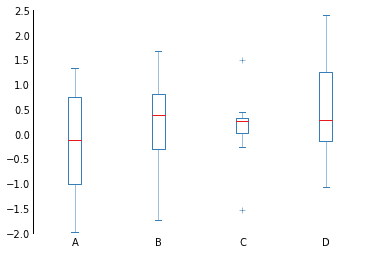Let's narrow the boxes a little, too.

plt.setp(bp['boxes'], color=set1, linewidth=0.5)
In :
import brewer2mpl
import random
import pandas as pd
import numpy as np

np.random.seed(10)

df = pd.DataFrame(np.random.randn(8, 4), columns=['A', 'B', 'C', 'D'])

set1 = brewer2mpl.get_map('Set1', 'qualitative', 7).mpl_colors

fig = plt.figure()

bp = ax.boxplot(df.values, widths=0.15)

ax.spines['top'].set_visible(False)
ax.spines['right'].set_visible(False)
ax.spines['bottom'].set_visible(False)

ax.xaxis.set_ticks_position('none')
ax.yaxis.set_ticks_position('none')

ax.xaxis.set_ticklabels(df.columns)

set1 = brewer2mpl.get_map('Set1', 'qualitative', 7).mpl_colors
plt.setp(bp['boxes'], color=set1, linewidth=0.5)
plt.setp(bp['medians'], color=set1)
plt.setp(bp['whiskers'], color=set1, linestyle='solid', linewidth=0.5)
plt.setp(bp['fliers'], color=set1)
plt.setp(bp['caps'], color=set1)

Out:
[None, None, None, None, None, None, None, None]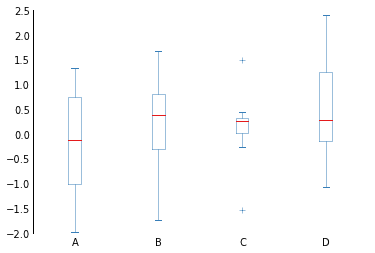Also, these caps aren't really adding that much. We can remove them and still have all the same information. We'll use this command,

plt.setp(bp['caps'], color='none')
In :
import brewer2mpl
import random
import pandas as pd
import numpy as np

np.random.seed(10)

df = pd.DataFrame(np.random.randn(8, 4), columns=['A', 'B', 'C', 'D'])

set1 = brewer2mpl.get_map('Set1', 'qualitative', 7).mpl_colors

fig = plt.figure()

bp = ax.boxplot(df.values, widths=0.15)

ax.spines['top'].set_visible(False)
ax.spines['right'].set_visible(False)
ax.spines['bottom'].set_visible(False)

ax.xaxis.set_ticks_position('none')
ax.yaxis.set_ticks_position('none')

ax.xaxis.set_ticklabels(df.columns)

set1 = brewer2mpl.get_map('Set1', 'qualitative', 7).mpl_colors
plt.setp(bp['boxes'], color=set1, linewidth=0.5)
plt.setp(bp['medians'], color=set1)
plt.setp(bp['whiskers'], color=set1, linestyle='solid', linewidth=0.5)
plt.setp(bp['fliers'], color=set1)
plt.setp(bp['caps'], color='none')

Out:
[None, None, None, None, None, None, None, None]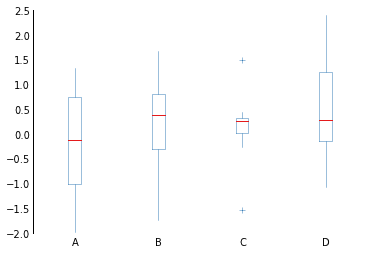Finally, let's narrow the axis on the left. It's a little tricky to access the linewidth of the 'spines', as there is no function that we can use to set it, so we'll have to access one of it's private variables using this command,

ax.spines['left']._linewidth = 0.5
In :
import brewer2mpl
import random
import pandas as pd
import numpy as np

np.random.seed(10)

df = pd.DataFrame(np.random.randn(8, 4), columns=['A', 'B', 'C', 'D'])

set1 = brewer2mpl.get_map('Set1', 'qualitative', 7).mpl_colors

fig = plt.figure()

bp = ax.boxplot(df.values, widths=0.15)
ax.xaxis.set_ticklabels(df.columns)

ax.spines['top'].set_visible(False)
ax.spines['right'].set_visible(False)
ax.spines['bottom'].set_visible(False)
ax.xaxis.set_ticks_position('none')
ax.yaxis.set_ticks_position('none')

set1 = brewer2mpl.get_map('Set1', 'qualitative', 7).mpl_colors
plt.setp(bp['boxes'], color=set1, linewidth=0.5)
plt.setp(bp['medians'], color=set1)
plt.setp(bp['whiskers'], color=set1, linestyle='solid', linewidth=0.5)
plt.setp(bp['fliers'], color=set1)
plt.setp(bp['caps'], color='none')

ax.spines['left']._linewidth = 0.5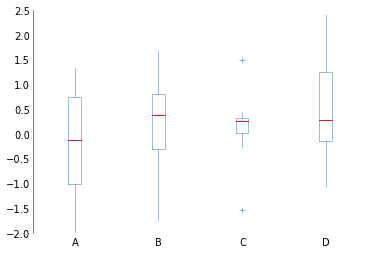## Sassy small multiples¶

A while ago I made this terrible diagram. The details are a little complicated, but here they are. The different colors are 13 different fission yeast cell cycle Schizosaccharomyces pombe (S. pombe) transcription factors (TFs), and their local sequence alignment score (Smith-Waterman, using Biopython's AlignIO) with 204 budding yeast Saccharomyces cerevisiae (S. cerevisae) TFs and their fraction common bound, which is a homebrewed metric of how many gene targets the pombe and cerevisiae TFs have in common.

This script /Users/olga/Dropbox/Docs/UCSD/rotations/q1-fall-ideker/src/YeastTFs.py has all the data we need to for this diagram.

In :
%run /Users/olga/Dropbox/Docs/UCSD/rotations/q1-fall-ideker/src/YeastTFs.py

In :
fig = plt.figure()

# Maximum value we see for fraction common bound
xlim = [0,0.25]

# Maximum value we see for alignment scores
ylim = [0,800]

feature='score'
alignment = 'local'

for i, tf in enumerate(yeast_tfs.pombe_tfs):
#    ax = axes[i]
ind = tf.binary_ind
x = tf.cerev_common_bound_fraction[tf.cerev_common_ind[
tf.cerev_common_ind != False]]
y = tf.__dict__['df_'+alignment].ix[ind,feature]

# Linear regression

ax.scatter(x, y, c=tf.color, edgecolor='black',
lw=0.2)
ax.set_ylim(ylim)
ax.set_xlim(xlim)

# Remove top and right axes and ticks
ax.spines['top'].set_visible(False)
ax.spines['right'].set_visible(False)
ax.yaxis.set_ticks_position('none')
ax.xaxis.set_ticks_position('none')

ax.set_title('Local alignment score vs Fraction common bound')

ax.set_xlabel('fraction common bound')
ax.set_ylabel('local alignment score')

plt.tight_layout()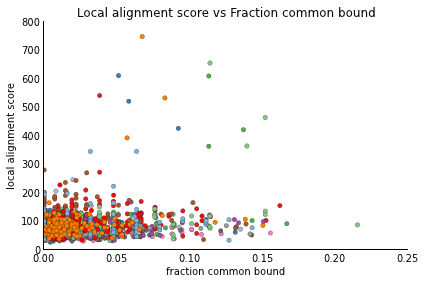I had good intentions, I used the right colors from ColorBrewer, I removed the right and top axes, there's no weird grid, but it's still really hard to interpret.

One of the major problems of this diagram is that there are way too many colors, and impossible to tell exactly which TF is different from the others. So instead of doing all those different colors on one plot, let's separate them all onto different plots. This technique is called small multiples, coined by Edward Tufte. It is an effective data presentation as the viewer is oriented to the axes of interest in the first plot, and since they understand the $x$- and $y$-axes of the first plot, they can understand the rest, and their eye can adjust to the tiny details that differ between them.

### Implementing small multiples¶

First we need to figure out how many plots we need to make. We know the number of TFs from the length of the yeast_tfs.pombe_tfs object. Then we'll take the square root of the length, and the ceiling of the square root, so we can fit all the plots onto one figure:

import math
num_plots = len(yeast_tfs.pombe_tfs)
n = int(math.ceil(math.sqrt(num_plots)))



Then we'll create many axes,

axes = [plt.subplot(n,n,i) for i in range(1,num_plots+1)]



And in iterate over them in the for loop,

ax = axes[i]



And we'll label each transcription factor's plot with its ID.

ax.set_title(tf.id)
In :
import math
num_plots = len(yeast_tfs.pombe_tfs)
n = int(math.ceil(math.sqrt(num_plots)))

fig = plt.figure(figsize=(10,10))

# Maximum value we see for fraction common bound
xlim = [0,0.25]

# Maximum value we see for alignment scores
ylim = [0,800]

axes = [plt.subplot(n,n,i) for i in range(1,num_plots+1)]
feature='score'
alignment = 'local'

for i, tf in enumerate(yeast_tfs.pombe_tfs):
ax = axes[i]
ind = tf.binary_ind
x = tf.cerev_common_bound_fraction[tf.cerev_common_ind[
tf.cerev_common_ind != False]]
y = tf.__dict__['df_'+alignment].ix[ind,feature]

ax.scatter(x, y, c=tf.color, edgecolor='black',
lw=0.2)
ax.set_ylim(ylim)
ax.set_xlim(xlim)

# Remove top and right axes and ticks
ax.spines['top'].set_visible(False)
ax.spines['right'].set_visible(False)
ax.yaxis.set_ticks_position('none')
ax.xaxis.set_ticks_position('none')

ax.set_title(tf.id)

ax.set_xlabel('fraction common bound')
ax.set_ylabel('local alignment score')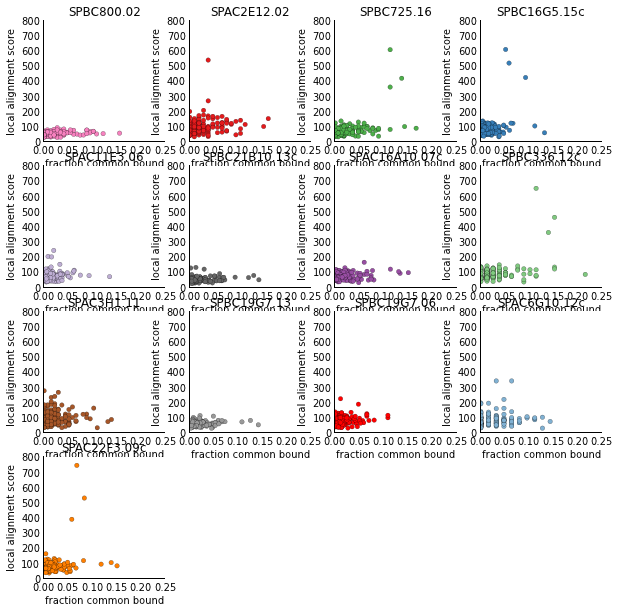This looks pretty terrible because of two main reasons:

1. The plot titles and axis labels are overlapping, which we can fix with plt.tight_layout()
2. The different colors aren't informative, they just clutter the diagram. We'll do this by fixing them all to one color.

We'll need to import brewer2mpl and use set2:

import brewer2mpl

# Need to specify a minimum of 3 colors to get any of the ColorBrewer colors
set2 = brewer2mpl.get_map('set2', 'qualitative', 3).mpl_colors
color = set2
In :
import math
num_plots = len(yeast_tfs.pombe_tfs)
n = int(math.ceil(math.sqrt(num_plots)))

import brewer2mpl
set2 = brewer2mpl.get_map('set2', 'qualitative', 3).mpl_colors
color = set2

fig = plt.figure(figsize=(10,10))

# Maximum value we see for fraction common bound
xlim = [0,0.25]

# Maximum value we see for alignment scores
ylim = [0,800]

axes = [plt.subplot(n,n,i) for i in range(1,num_plots+1)]
alignments = ['local', 'global']
features = ['identity', 'similarity', 'score']

feature='score'

alignment = 'local'
for i, tf in enumerate(yeast_tfs.pombe_tfs):
ax = axes[i]
ind = tf.binary_ind
x = tf.cerev_common_bound_fraction[tf.cerev_common_ind[
tf.cerev_common_ind != False]]
y = tf.__dict__['df_'+alignment].ix[ind,feature]

ax.scatter(x, y, c=color, edgecolor='black',
lw=0.2)
ax.set_ylim(ylim)
ax.set_xlim(xlim)

# Remove top and right axes and ticks
ax.spines['top'].set_visible(False)
ax.spines['right'].set_visible(False)
ax.yaxis.set_ticks_position('none')
ax.xaxis.set_ticks_position('none')

ax.set_title(tf.id)

ax.set_xlabel('fraction common bound')
ax.set_ylabel('local alignment score')

plt.tight_layout()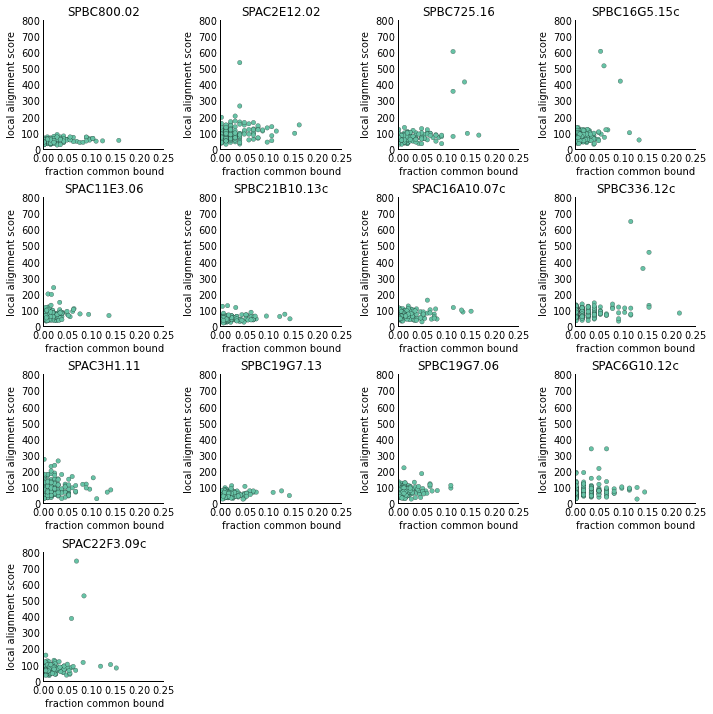This is great, but what would make this figure even better is a linear regression line, which would show easy-to-spot patterns among the different transcription factors.

from scipy import stats
...
# Linear regression
slope, intercept, r_value, p_value, std_err = stats.linregress(x,y)
x_fit = np.arange(xlim, xlim, (xlim-xlim)/100.)
y_fit = np.multiply(x_fit, slope) + intercept

# Plot linear regression line
ax.plot(x_fit,y_fit, color='grey')

# Write 'R' value onto plot
ax.text(xlim, ylim,'$R^2$ = %.4f' % r_value,fontsize=12,
ha='right',va='top')
In :
import math
num_plots = len(yeast_tfs.pombe_tfs)
n = int(math.ceil(math.sqrt(num_plots)))

from scipy import stats

import brewer2mpl
set2 = brewer2mpl.get_map('set2', 'qualitative', 3).mpl_colors
color = set2

fig = plt.figure(figsize=(10,10))

# Maximum value we see for fraction common bound
xlim = [0,0.25]

# Maximum value we see for alignment scores
ylim = [0,800]

axes = [plt.subplot(n,n,i) for i in range(1,num_plots+1)]
alignments = ['local', 'global']
features = ['identity', 'similarity', 'score']

feature='score'

alignment = 'local'
for i, tf in enumerate(yeast_tfs.pombe_tfs):
ax = axes[i]
ind = tf.binary_ind
x = tf.cerev_common_bound_fraction[tf.cerev_common_ind[
tf.cerev_common_ind != False]]
y = tf.__dict__['df_'+alignment].ix[ind,feature]

ax.scatter(x, y, c=color, edgecolor='black',
lw=0.2)
ax.set_ylim(ylim)
ax.set_xlim(xlim)

# Remove top and right axes and ticks
ax.spines['top'].set_visible(False)
ax.spines['right'].set_visible(False)
ax.yaxis.set_ticks_position('none')
ax.xaxis.set_ticks_position('none')

ax.set_title(tf.id)

ax.set_xlabel('fraction common bound')
ax.set_ylabel('local alignment score')

# Linear regression
slope, intercept, r_value, p_value, std_err = stats.linregress(x,y)
x_fit = np.arange(xlim, xlim, (xlim-xlim)/100.)
y_fit = np.multiply(x_fit, slope) + intercept

# Plot linear regression line
ax.plot(x_fit,y_fit, color='grey')

# Write 'R' value onto plot
ax.text(xlim, ylim,'$R^2$ = %.4f' % r_value,fontsize=12,
ha='right',va='top')

plt.tight_layout()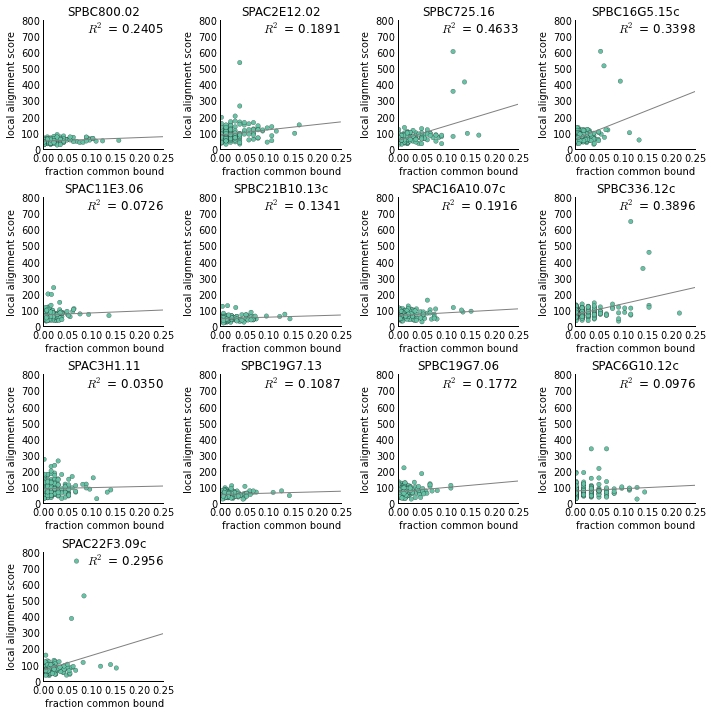Finally, let's thin out the axis lines as we did with the boxplot, with

ax.spines['left']._linewidth = 0.5
ax.spines['bottom']._linewidth = 0.5
In :
import math
num_plots = len(yeast_tfs.pombe_tfs)
n = int(math.ceil(math.sqrt(num_plots)))

from scipy import stats

import brewer2mpl
set2 = brewer2mpl.get_map('set2', 'qualitative', 3).mpl_colors
color = set2

fig = plt.figure(figsize=(10,10))

# Maximum value we see for fraction common bound
xlim = [0,0.25]

# Maximum value we see for alignment scores
ylim = [0,800]

axes = [plt.subplot(n,n,i) for i in range(1,num_plots+1)]
alignments = ['local', 'global']
features = ['identity', 'similarity', 'score']

feature='score'

alignment = 'local'
for i, tf in enumerate(yeast_tfs.pombe_tfs):
ax = axes[i]
ind = tf.binary_ind
x = tf.cerev_common_bound_fraction[tf.cerev_common_ind[
tf.cerev_common_ind != False]]
y = tf.__dict__['df_'+alignment].ix[ind,feature]

ax.scatter(x, y, c=color, edgecolor='black',
lw=0.2)
ax.set_ylim(ylim)
ax.set_xlim(xlim)

# Remove top and right axes and ticks
ax.spines['top'].set_visible(False)
ax.spines['right'].set_visible(False)
ax.yaxis.set_ticks_position('none')
ax.xaxis.set_ticks_position('none')

ax.set_title(tf.id)

ax.set_xlabel('fraction common bound')
ax.set_ylabel('local alignment score')

# --- Linear regression --- #
slope, intercept, r_value, p_value, std_err = stats.linregress(x,y)
x_fit = np.arange(xlim, xlim, (xlim-xlim)/100.)
y_fit = np.multiply(x_fit, slope) + intercept

# Plot linear regression line
ax.plot(x_fit,y_fit, color='grey')

# Write 'R' value onto plot
ax.text(xlim, ylim,'$R^2$ = %.4f' % r_value,fontsize=12,
ha='right',va='top')

# --- Thin out the axis lines --- #
ax.spines['left']._linewidth = 0.5
ax.spines['bottom']._linewidth = 0.5

plt.tight_layout()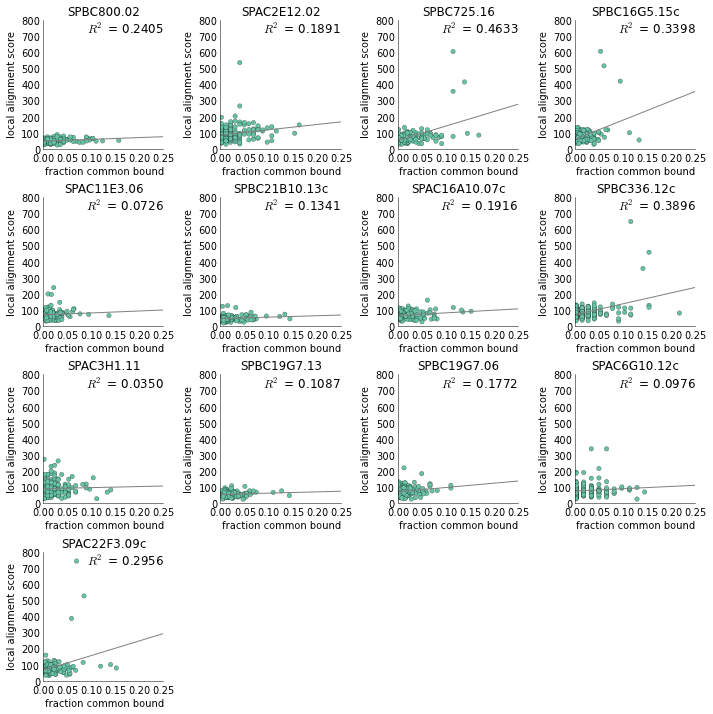And actually now that I think about it, we really only need to label one of the axes since all the x- and y- axes are the same, just different TFs. We'll do that by:

for i, tf in enumerate(yeast_tfs.pombe_tfs):
...
if i == 0:
ax.set_xlabel('fraction common bound')
ax.set_ylabel('local alignment score')
In :
import math
num_plots = len(yeast_tfs.pombe_tfs)
n = int(math.ceil(math.sqrt(num_plots)))

from scipy import stats

import brewer2mpl
set2 = brewer2mpl.get_map('set2', 'qualitative', 3).mpl_colors
color = set2

fig = plt.figure(figsize=(10,10))

# Maximum value we see for fraction common bound
xlim = [0,0.25]

# Maximum value we see for alignment scores
ylim = [0,800]

axes = [plt.subplot(n,n,i) for i in range(1,num_plots+1)]
alignments = ['local', 'global']
features = ['identity', 'similarity', 'score']

feature='score'

alignment = 'local'
for i, tf in enumerate(yeast_tfs.pombe_tfs):
ax = axes[i]
ind = tf.binary_ind
x = tf.cerev_common_bound_fraction[tf.cerev_common_ind[
tf.cerev_common_ind != False]]
y = tf.__dict__['df_'+alignment].ix[ind,feature]

ax.scatter(x, y, c=color, edgecolor='black',
lw=0.2)
ax.set_ylim(ylim)
ax.set_xlim(xlim)

ax.set_title(tf.id)

# Remove top and right axes and ticks
ax.spines['top'].set_visible(False)
ax.spines['right'].set_visible(False)
ax.yaxis.set_ticks_position('none')
ax.xaxis.set_ticks_position('none')

# --- Linear regression --- #
slope, intercept, r_value, p_value, std_err = stats.linregress(x,y)
x_fit = np.arange(xlim, xlim, (xlim-xlim)/100.)
y_fit = np.multiply(x_fit, slope) + intercept

# Plot linear regression line
ax.plot(x_fit,y_fit, color='grey')

# Write 'R' value onto plot
ax.text(xlim, ylim,'$R^2$ = %.4f' % r_value,fontsize=12,
ha='right',va='top')

# --- Thin out the axis lines --- #
ax.spines['left']._linewidth = 0.5
ax.spines['bottom']._linewidth = 0.5

# Only label the x and y axis of the first plot, since they are all the same
if i == 0:
ax.set_xlabel('fraction common bound')
ax.set_ylabel('local alignment score')

plt.tight_layout()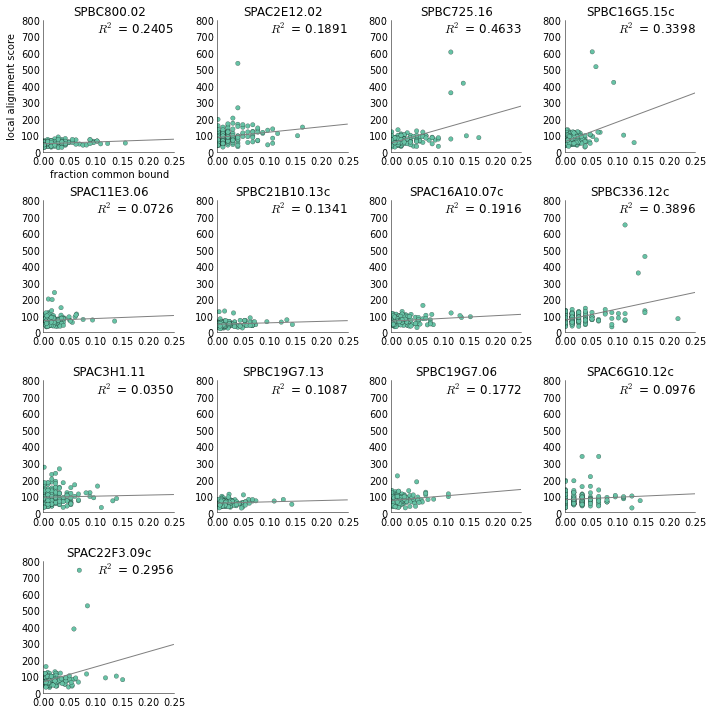Now this looks nice. Let's clean up the tick labels a little, because it still looks cluttered around the axes. We'll remove a portion of the ticks using,

# Python's built-in range is fine for making a sequence of integers
ax.yaxis.set_ticks(range(ylim/4,ylim+ylim/4,ylim/4))

# Need to use np.arange to make a sequence of floats
ax.xaxis.set_ticks(np.arange(xlim/5, xlim+2*xlim/5, 2*xlim/5))
In :
import math
num_plots = len(yeast_tfs.pombe_tfs)
n = int(math.ceil(math.sqrt(num_plots)))

from scipy import stats

import brewer2mpl
set2 = brewer2mpl.get_map('set2', 'qualitative', 3).mpl_colors
color = set2

fig = plt.figure(figsize=(10,10))

# Maximum value we see for fraction common bound
xlim = [0,0.25]

# Maximum value we see for alignment scores
ylim = [0,800]

axes = [plt.subplot(n,n,i) for i in range(1,num_plots+1)]
alignments = ['local', 'global']
features = ['identity', 'similarity', 'score']

feature='score'

alignment = 'local'
for i, tf in enumerate(yeast_tfs.pombe_tfs):
ax = axes[i]
ind = tf.binary_ind
x = tf.cerev_common_bound_fraction[tf.cerev_common_ind[
tf.cerev_common_ind != False]]
y = tf.__dict__['df_'+alignment].ix[ind,feature]

ax.scatter(x, y, c=color, edgecolor='black',
lw=0.2)
ax.set_ylim(ylim)
ax.set_xlim(xlim)

ax.set_title(tf.id)

# Remove top and right axes and ticks
ax.spines['top'].set_visible(False)
ax.spines['right'].set_visible(False)
ax.yaxis.set_ticks_position('none')
ax.xaxis.set_ticks_position('none')

ax.spines['left']._linewidth = 0.5
ax.spines['bottom']._linewidth = 0.5

# --- Linear regression --- #
slope, intercept, r_value, p_value, std_err = stats.linregress(x,y)
x_fit = np.arange(xlim, xlim, (xlim-xlim)/100.)
y_fit = np.multiply(x_fit, slope) + intercept

# Plot linear regression line
ax.plot(x_fit,y_fit, color='grey')

# Write 'R' value onto plot
ax.text(xlim, ylim,'$R^2$ = %.4f' % r_value,fontsize=12,
ha='right',va='top')

# --- Thin out the axis lines --- #
ax.spines['left']._linewidth = 0.5
ax.spines['bottom']._linewidth = 0.5

# Only label the x and y axis of the first plot, since they are all the same
if i == 0:
ax.set_xlabel('fraction common bound')
ax.set_ylabel('local alignment score')

# Python's built-in range is fine for making a sequence of integers
ax.yaxis.set_ticks(range(ylim/4,ylim+ylim/4,ylim/4))

# Need to use np.arange to make a sequence of floats
ax.xaxis.set_ticks(np.arange(xlim/5, xlim+2*xlim/5, 2*xlim/5))

plt.tight_layout()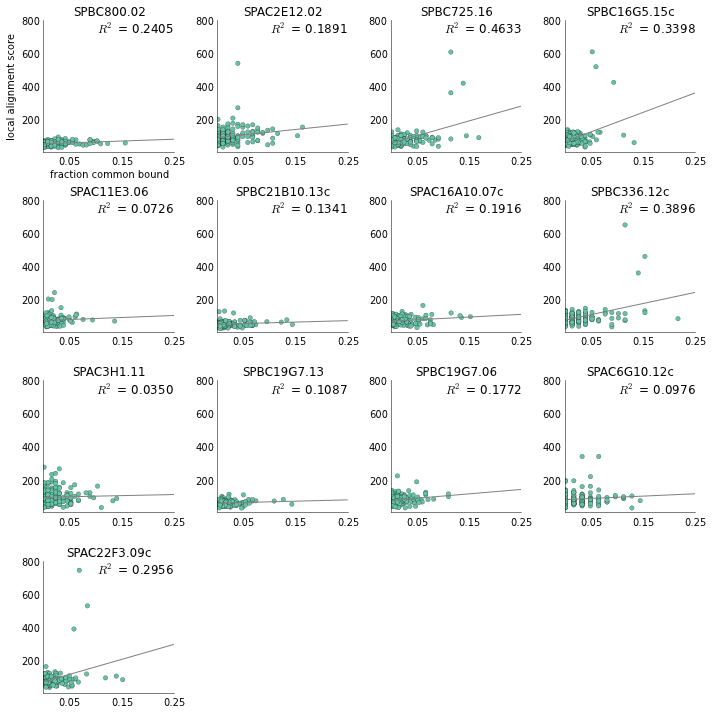Now this looks great! And it's easy to see that transcription factors SPAC2E12.02, SPBC725.16, SPBC16G5.15c, SPBC336.12c, SPAC6G10.12c, and SPAC22F3.09c are more "interesting" than the others, since they have more outliers from their fitted line.

## Conclusions¶

The main takeaways from this tutorial are:

• Boxplots are good, but need some adjustment so they're easier on the eyes
• Small multiples are an effective method to tease apart complex, multidimensional datasets
In [ ]: